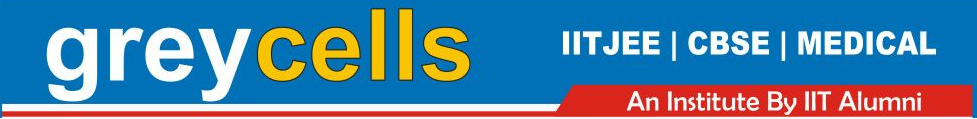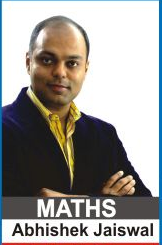Maths Lectures by Abhishek Jaiswal (B.Tech IIT Roorkee) for class 12th Students. The lectures cover theory both for CBSE and Competetive Examinations.

For Queries and Live online batches contact 9312922770.Lecture 1 | Vectors | Introduction | Direction Cosines

Lecture 2 | Vectors | Addition and Subtraction of Vectors

Lecture 3 | Vectors | Collinearity & Coplanarity of Vectors

Lecture 4 - Question Solving | Vectors

Lecture 5 | Vectors - Dot/Scalar Product

Lecture 6 | Vectors - Questions on Dot Product

Lecture 7 | Vectors - Product of Vectors

Lecture 8 | Vectors - Vector/Cross Product of Vectors

Lecture 8 | PART 2 | Vectors - Vector/Cross Product of Vectors

Lecture 9 | Vectors - Product of Vectors | Scalar Triple Product

Lecture 10 | Vectors - Scalar Triple Product

Lecture 11 | Vectors - Vector Triple Product

Lecture 12 | Vectors - Vector Equation and Introduction to 3D